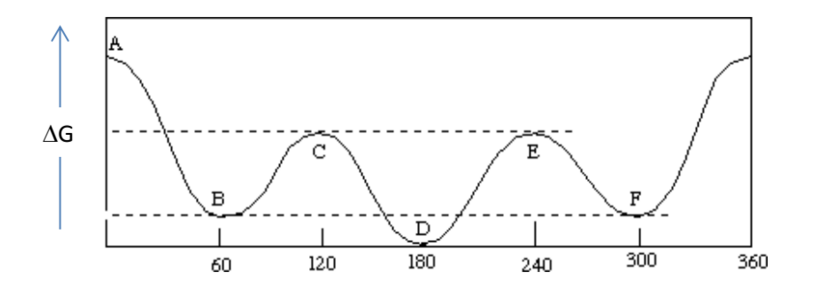# Problem: The following chart describes the relationship between potential energy and torsional angle in butane (the torsional angle being measured about the C2-C3 bond). Identify the labeled maxima or minima as representing fully eclipsed, gauche or anti conformer energies. NOTE: "anti" or "trans" have the same definition: the groups are away from each other at a 180° angle.

###### FREE Expert Solution
91% (158 ratings)
###### Problem Details

The following chart describes the relationship between potential energy and torsional angle in butane (the torsional angle being measured about the C2-C3 bond). Identify the labeled maxima or minima as representing fully eclipsed, gauche or anti conformer energies. NOTE: "anti" or "trans" have the same definition: the groups are away from each other at a 180° angle.What scientific concept do you need to know in order to solve this problem?

Our tutors have indicated that to solve this problem you will need to apply the Dihedral Angle concept. If you need more Dihedral Angle practice, you can also practice Dihedral Angle practice problems.

What is the difficulty of this problem?

Our tutors rated the difficulty ofThe following chart describes the relationship between poten...as high difficulty.

How long does this problem take to solve?

Our expert Organic tutor, Chris took 4 minutes and 23 seconds to solve this problem. You can follow their steps in the video explanation above.

What professor is this problem relevant for?

Based on our data, we think this problem is relevant for Professor de St Germain's class at FAU.